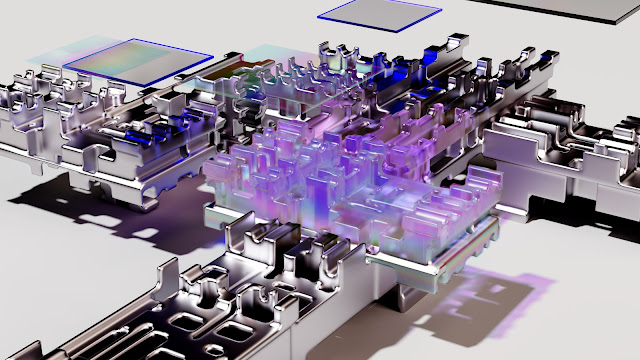# Deep Learning - Convolutional Neural Networks in 2023

Convolutional Neural Network (CNN) is a deep leaming neural network designed for processing structured arrays of data such as images. A CNN is a feed-forward neural network, often with up to 20 or 30 layers.

Convolutional neural network is also called ConvNet. multiplied to provide an output that is used to extract information from the image.

• In CNN, 'convolution' is referred to as the mathematical function. It's a type of linear expresses how one function's shape can be changed by the other.

• The goal of CNN is to the images so that it would be easier to process without losing features.

• A convolutional neural network is made up of numerous layers, such as convolution layers, pooling layers and fully connected layers and it uses a back-propagation algorithm to learn spatial hierarchies of data automatically and adaptively. To understanding the Concepts of Convolutional Neural Networks (CNNs) an example of the images our brain can be interpret.• As soon as we see an image, our brain starts categorizing it based on the color, shape and sometimes also the message that image is conveying. Similar thing can be done through machines even after a rigorous training. But the difficulty is there is a huge difference in what humans interpret and what machine does. For a machine, the image is merely an array of pixels. There is a unique pattern included, the image is merely an array pixels. There is a unique pattern included in each object present in the image and the computer tries to find out these patterns to get the information about the image.

Machines can be trained giving tons of images to increase its ability to recognize the objects included in a given input image.

Most of the digital companies have opted for CNNs for image recognition, some of these include Google, Amazon, Instagram, Interest, Facebook, etc, multiple convolutional layers which are used mainly for image processing. classification, segmentation and other correlated data".

• CNN automatically detects the important features without any human supervision.

• CNN is also computationally efficient.

• Higher accuracy.

• Weight sharing is another major advantage of CNNs.

• CNNs make use of the same knowledge across all image locations.

• Adversarial attacks are cases of feeding the network 'bad' examples to cause misclassification.

• CNN requires lot of training data.

Application of CNN:

• CNN is mostly used for image classification, for example to determine the satellite images containing mountains and valleys or recognition of handwriting, etc. image segmentation, processing, etc. are the areas where CNN are used. probable visual image to represent that data.

• Voice synthesis: Google Assistant's voice synthesizer uses Deepmind's WaveNet Astrophysics: They are used to make sense of radio telescope data.

• A convolutional neural network, as discussed above, has the following layers that useful for various deep learning algorithm. Let us see the working of these laym taking an example of the image having dimension of 12 x 12 x 4. These are: 1. Imput layer: This layer will accept the image of width 12, height 12 and depth 4.

Convolution layer:

It computes the volume of the image by getting the dot product between the image filters possible and the image patch. For example, there are 10 filters possible, then the volume will be computed as 12 x 12 x 10. 3. Activation function layer: This layer applies activation function to each element in the output of the convolutional layer. Some of the well accepted activation functions are ReLu, Sigmoid, Tank Leaky Relu, ez. These function will not change the volume obtained at the convolutional layer and hence it will remain equal to 12 x 12 x 10.

Pool layer:

This function mainly reduces the volume of the intermediate outputs, which enables fast computation of the network model, thus preventing it from overfitting.

Convolution Operation:

Convolution operation allows the network to detect horizontal and vertical edges of an image and then based on those edges build high-level features in the following layers of neural network.

In general form, convolution is an operation on two functions of a real valued argument To motivate the definition of convolution, we start with examples of two functions.

Suppose we are tracking the location of a spaceship with a laser sensor Laser sensor des a single output x(0), the position of the spaceship at time t.

To obtain a less noisy estimate of the spaceship's position, we would like to average together several measurements Of , more recent measurements are more relevant, so we will want this to he weighted average that gives more weight to recent measurements.

We can do this with a weighting function w(a), where "a" is the age of a measurement If we apply such a weighted average operation at every moment, we obtain a new function providing a smoothed estimate of the position "s" of the spaceship Convolution operation uses three parameters: Input image, detector.